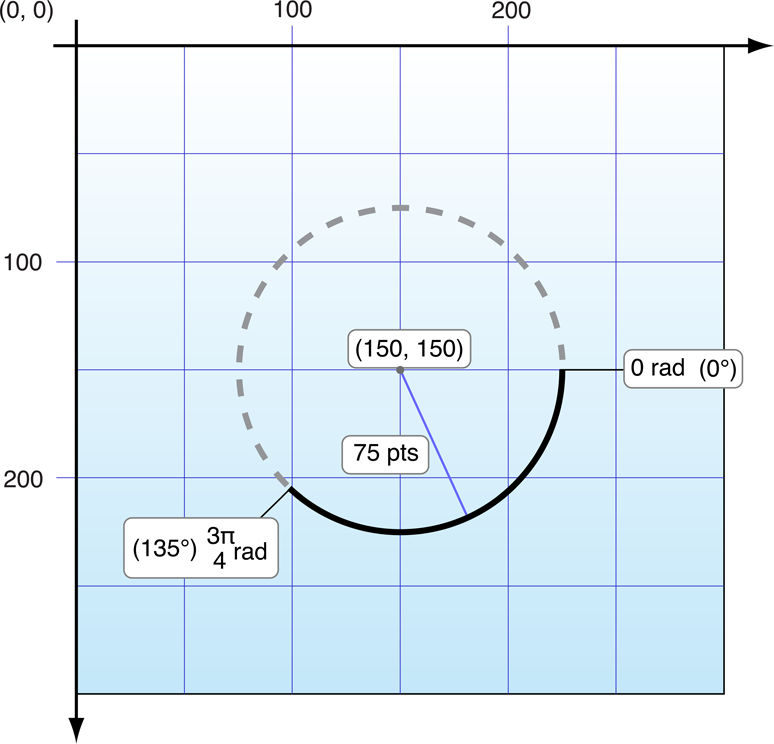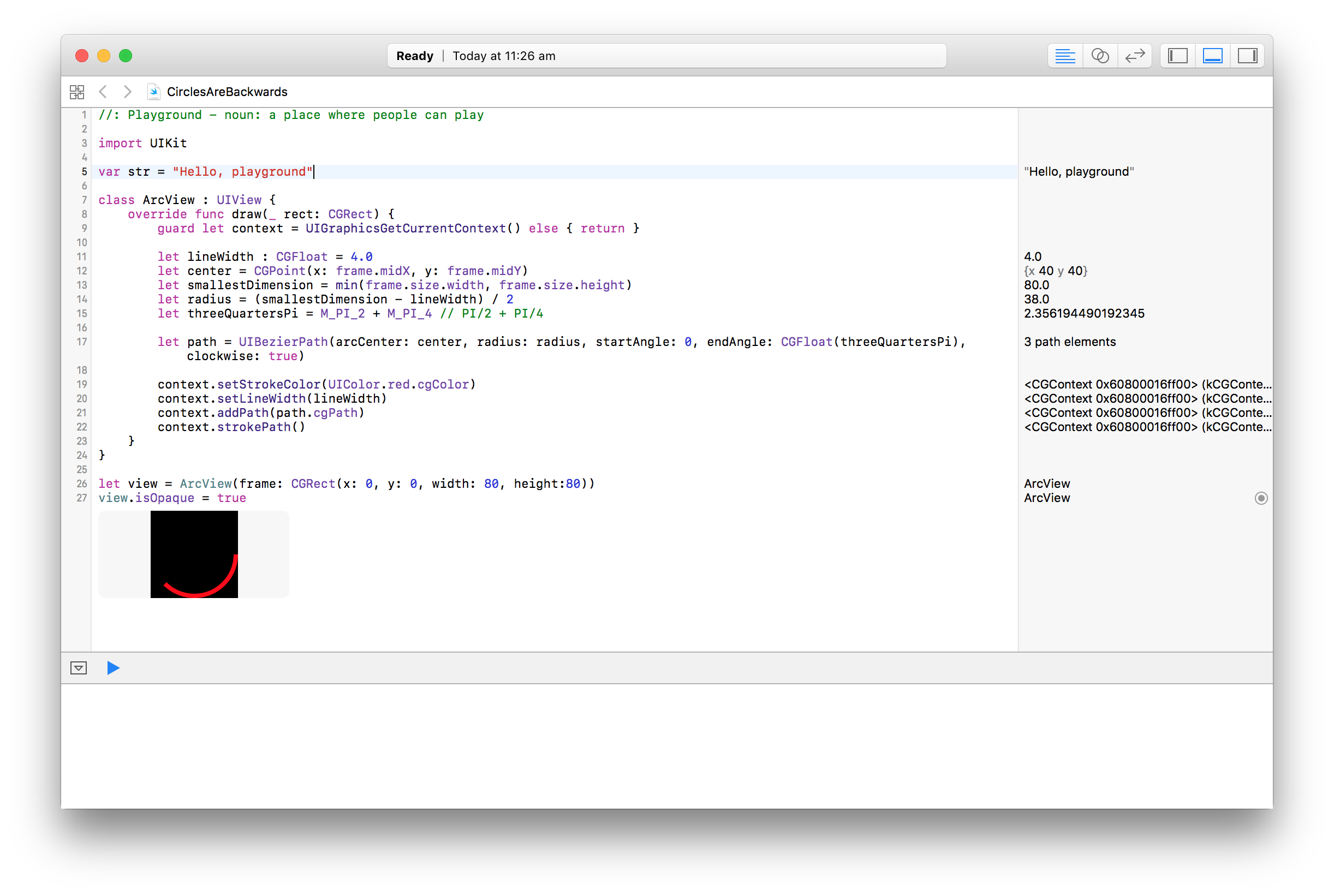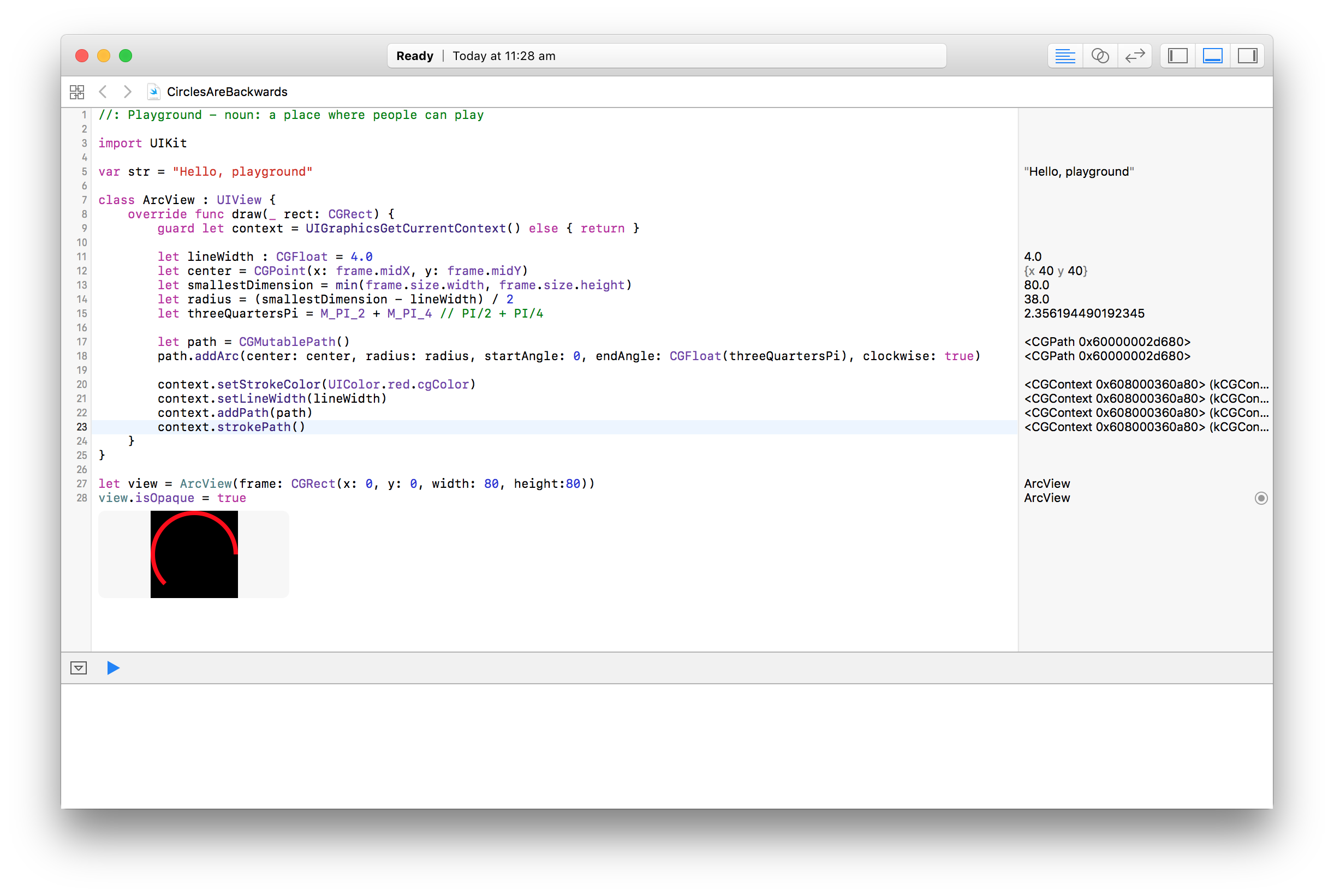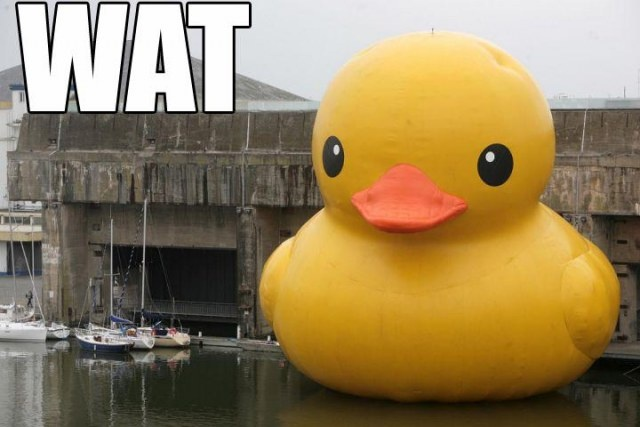Let's say we want to draw a custom view on iOS. This is usually where you we jump straight into Core Graphics and override `- (void) drawRect:`, or in Swift 3, `draw(_ rect: CGRect)`.

(I'm going to use Swift 3 for this blog post as this is what I was using when I stumbled across this issue, as a learning exercise. I'm sorry if this blog post no longer compiles in another 9 months time.)

I've discussed it at length with other developers and looked at it upside down, but I'm 100% certain that iOS is drawing arcs backwards.

Let's start by looking at the foundations here provided by Core Graphics. The first thing we need to understand is the coordinate system.

On macOS, Core Graphics uses a coordinate system starting at the lower-left of the screen. On iOS, Core Graphics uses a coordinate system starting at the upper-left of the screen. This is well-defined by Apple's documentation:

Each of the drawing frameworks of iOS establishes a default coordinate system based on the current graphics context. In iOS, there are two main types of coordinate systems:

• An upper-left-origin coordinate system (ULO), in which the origin of drawing operations is at the upper-left corner of the drawing area, with positive values extending downward and to the right. The default coordinate system used by the UIKit and Core Animation frameworks is ULO-based.
• A lower-left-origin coordinate system (LLO), in which the origin of drawing operations is at the lower-left corner of the drawing area, with positive values extending upward and to the right. The default coordinate system used by Core Graphics framework is LLO-based.

The second thing we need to understand is how circles and arcs are drawn. Again, this is well-defined by Apple's documentation as being in radians, measured clockwise, with 0 being the right-hand side of the circle, as shown by Figure 2-2.Furthermore, immediately above this diagram, Apple state that:

In this case, the arc is created in the clockwise direction. (Drawing the arc in the counterclockwise direction would paint the dashed portion of the circle instead.)

The sample code that Apple provide uses the value of `0` for the start angle, `DEGREES_TO_RADIANS(135)` for the end angle, and `YES` for `clockwise` (in Objective-C).

We can replicate this perfectly in a custom view by drawing with those same values:However, if we replace `UIBezierPath` with a custom-built `CGPath`, things go backwards:The only way to get the `CGPath` path to match the `UIBezierPath` path is to either:

• Switch the `startAngle` and `endAngle` values, or
• Set `clockwise` to `false` instead of `true`

Without doing either of those, or by doing both, Core Graphics either draws clockwise from the end angle to the start angle, or it draws anti-clockwise from the start angle to the end angle.

To quote Gary Bernhardt:I've uploaded a playground you can use to play around with the values, but there are only two options I can see where any of this makes sense:

1. `UIBezierPath` measures angles clockwise from the right-hand side (0 degrees) and draws correctly. `CGPath` flips the canvas upside-down by the Y axis, measures angles anti-clockwise from the right-hand side (0 degrees), draws clockwise (or anti-clockwise, if instructed to), then flips the canvas again. If this is the case, this API is completely counter-intuitive.

2. CGPath just draws the circles backwards.

If anyone from Apple is reading this, this has been filed as Radar 28579645.

1. iOS Drawing Concepts -  Developer ↩︎

2. Drawing Shapes Using Bézier Paths -  Developer ↩︎

3. Wat - Destroy All Software ↩︎